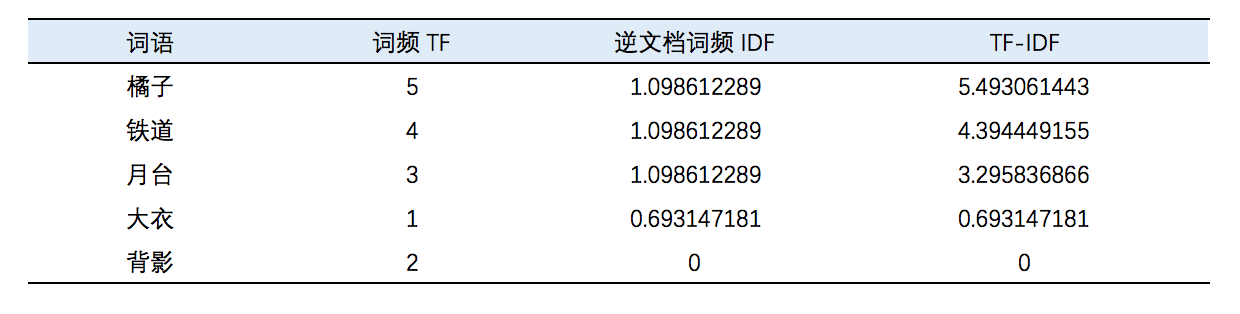## 原理部分

$$TF = 某个词在文档中的出现次数$$

$$IDF = log(\frac{语料库中文档的总数}{语料库中包含该词语的文档数+1})$$

$$TF-IDF_i = TF_i \times IDF_i$$## Java实现部分

Java部分我们需要使用到Ansj分词器进行分词

Ansj分词器的Github：传送门

eval()方法的执行流程是这样的

Notes 注意了